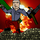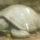## Social Question# What do you think of this proof of trigonometric angle addition formula?

Asked by LostInParadise (28580) May 6th, 2011

I am surely not the first to think of this, but this proof seems more intuitve and straightforward than the usual proofs I have seen using similar triangles. Angle Addition proof I give the proof for the cosine formula. The same diagram can be used for the sine formula.

Observing members: 0Composing members: 0Seems intuitive enough, but the first statement, that vector addition is independent of the coordinate frame, seems like a conclusion that might have been reached with the help of trigonometric angle addition. If so, this reasoning is circular. Nevertheless, it would be a good teaching method if not.

In short, did they use preexisting trigonometric angle addition in the background of this proof?

jasonwiese55 (620)“Great Answer” (1) Flag as…Vector addition uses parallelogram completion. It does not require a coordinate system.

LostInParadise (28580)“Great Answer” (0) Flag as…@LostInParadise Then it seems good to me.

jasonwiese55 (620)“Great Answer” (0) Flag as…Its a nice proof, but very hard to follow in its current form.

roundsquare (5517)“Great Answer” (0) Flag as…